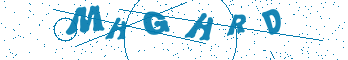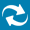# Enhancing Verilog Testbench Using Matlab® Interface

## Introduction

The Matlab interface was introduced in its full version in Active-HDL 7.1. If you are using earlier versions, only Simulink interface is available. The key difference between those two interfaces is that in Matlab interface Active-HDL controls the co-simulation process and starts Matlab when necessary, while in Simulink interface the MathWorks Simulink controls the co-simulation process and starts Active-HDL when necessary.

The Matlab interface, available from Verilog code level, provides the following features:

• Running of Matlab commands (both native and user-created m-files)

• Passing variables between Active-HDL and Matlab

• Creation and destruction of data structures required for arrays transfer between Active-HDL and Matlab

• Quick array data passing between Active-HDL and Matlab

Typical tasks that can be accomplished thanks to the Matlab interface include:

• Computation of functions hard to describe in HDLs

• Quick generation of complicated waveforms

• Post-processing of simulation results

• Advanced visualization of simulation results

To fully utilize the interface, you have to have Matlab version 7 (R14) or later installed on your computer and registered as a COM server ( type matlab /regserver in the command prompt to perform the registration).

To use Matlab interface, you must call special functions and tasks from your Verilog code (Matlab windows will show up after the first command or data transfer request is executed). To make those external routines visible in your code, you have to configure your environment. Matlab windows will show up after the first command or data transfer request is executed.

All modules that have to access Matlab interfaces must be compiled with link to the PLI library link2ml.dll located in the BIN subfolder of the Active-HDL installation. If you are using GUI to compile and run simulations, go to Design | Settings | Verilog PLI Application and point to the aldec_matlab_cosim.dll file. If you are compiling and initializing simulation from the .do script, remember to add the following switch to compilation (alog) and simulation (asim) commands:

```	-pli "\$ALDEC/BIN/aldec_matlab_cosim.dll"
```

## Importing Data from Matlab

The simplest task frequently useful in testbenches is generation of a waveform in Matlab and import of waveform data to the testbench. In the examples that follow we assume that appropriate libraries were already attached and made visible to your testbench code.

Our task is to generate modulate sine wave stimulus with the following parameters:

• Carrier frequency CFRQ equal 40

• Carrier amplitude AMPL equal 16383

• Signal frequency SFRQ equal 4

• Modulation depth DPTH equal 0.8

The mathematical formula describing the stimulus is:

```	AMPL*sin(2*pi*CFRQ*t) * (1-DPTH*cos(2*pi*SFRQ*t))
```

Assuming sampling period 1 ns, we want to create at least 1000 samples in Matlab, collect them in an array, transfer the array to the Active-HDL and use it to generate waveform fed to the 16 bit input DI of a test circuit (e.g. digital filter).

To generate samples in Matlab, we have to create a do_wave.m file in the SRC folder of your Active-HDL project and type two lines in that file:

```	t = 0:.001:1.024 ;
sw = fix(AMPL*sin(2*pi*CFRQ*t).*(1-DPTH*cos(2*pi*SFRQ*t))) ;
```

Notes:

• The first line creates time domain vector t, storing all values between 0 and 1.024 with 0.001 step

• The second line creates our waveform

• The Matlab function fix rounds its argument to the nearest integer towards zero

• The Matlab operator .* multiplies elements of both sine waves (regular * operator would attempt matrix multiplication in this context)

Please refer to Matlab documentation for more detailed description of Matlab functions.

To distinguish between row and column structures, one dimensional arrays in Matlab are internally stored as two dimensional arrays: 1-by-n for row-vectors and n-by-1 for column-vectors. This distinction is reflected in the internal structure of both t and sw arrays: they are two-dimensional arrays, with the first dimension size equal 1, and the second dimension size equal 1025. This structure is maintained after import to the Active-HDL environment.

The following variable declarations should be added to the Verilog testbench:

```	integer inp_arr_id;
reg signed [15:0] stim_arr [1:1025];
```

Notes:

• inp_arr_id will hold the id of array of samples imported from Matlab

• stim_arr is an array (memory) storing samples for waveform generation in Verilog

To pass values of stimulus generation parameters to Matlab, you have to enable \$put_variable task from the aldec_matlab_cosim.dll PLI library:

```	\$put_variable("AMPL", amplitude);
\$put_variable("CFRQ", carrier_frq);
\$put_variable("SFRQ", signal_frq);
\$put_variable("DPTH", mod_depth);
```

The first argument of the task is the string representing Matlab variable name. The second argument is value that should be assigned to that variable (we are using Verilog variables as the second argument).

HINT: The first use of Matlab-related procedures during simulation starts Matlab application. On some installations of Microsoft Windows loading Matlab for the first time is quite long. To avoid this period of apparent inactivity during simulation, you may consider starting and immediately closing Matlab before you run simulation in Active-HDL. Subsequent runs of Matlab should be faster.

To execute the m-file presented earlier and import the sw array created in Matlab, you have to enable a task and call a function from the aldec_matlab_cosim.dll PLI library:

```	\$eval_string( "do_wave" );
inp_arr_id = \$ml2hdl( "sw" );
```

Notes:

• \$eval_string task executes Matlab command or m-file passed as its string argument

• \$ml2hdl function imports Matlab array passed as its string argument and returns integer identifier for the imported array

As we have mentioned before, the sw array maintains its original 1-by-1025 size after import. That's why we have to use the following syntax of the \$get_item task to transfer the elements from the imported array to the regular, Verilog array variable stim_arr:

```	for ( i=1; i<1026; i=i+1) begin : m2v
\$get_item( stim_arr[i], 0, inp_arr_id, 1, i );
end
```

Notes:

• stim_arr[i] is the memory cell where one element of the imported array will be stored

• 0 is the number of digits in the fractional part of the value being transferred

• inp_arr_id is the identifier of the imported array (returned by \$ml2hdl)

• 1 and i are indices of the position of the transferred element in the imported array

To complete our task, we have to transfer samples stored in the stim_arr memory to the input of the tested circuit:

```	for ( i=1; i<1026; i=i+1) begin : v2p
DI = stim_arr[i];
#1;
end
\$destroy_array( inp_arr_id );
```

Note:

• \$destroy_array task cleans up the memory allocated for the imported array.

## Exporting Data to Matlab

Waveform viewer in your HDL simulator is powerful, but restricted to time-domain graphs (waveforms). If you want to display any other graphs (e.g. Fast Fourier Transform of the data produced by the tested circuit), Matlab is an excellent solution.

In this section of the note, our task is to:

• collect 512 samples of the output DO of our tested circuit,

• create temporary 1-by-512 array required for export procedures

• transfer samples to the temporary array

• export temporary array to Matlab

• process and graph exported data

• import some computation results back to the HDL simulator

To tell Matlab how to process exported data we have to create m-file named do_fft.m in our design and fill it with Matlab commands computing FFT and creating graph. Here's the list of Matlab commands:

```	Y=fft(smpl,512) ;
My = sqrt(Y.* conj(Y)) ;
f = 1000*(0:256)/512 ;
[MM, MI] = max( My(1:50) );
MF = f(MI);
plot(f,My(1:257))
title('Frequency content of filter output')
xlabel('frequency (Hz)')
grid on
```

Notes:

• the first line requests computation of 512 point FFT and stores results in complex matrix Y

• the second line computes modules of FFT coefficients stored in Y and sends them to My matrix

• the third line computes frequency domain required for our final graph; since the results of FFT are symmetrical, we only have to use half of the points

• the fourth and fifth line find maximal power frequency in the previously computed spectrum of the output signal

• the remaining lines create graph, label it and its frequency axis, display grid

You will need an array to store samples of the output of the tested circuit. Here?s an example of the variable declaration:

```	reg [15:0] samples [1:512];
```

You should write appropriate sampling code, according to the nature of the tested circuit and your verification procedures. The only assumption we are making is the sampling period of 1 ns. Once the samples array is filled with data, we can proceed to the export to Matlab:

```	out_arr_id = \$create_array( "smpl", 1, 512); // create temp. array
// transfer samples to temp. array:
for ( i=1; i<513; i=i+1)
\$put_item( samples[i], 0, out_arr_id, 1, i );
\$hdl2ml( out_arr_id );                 // send temp. array to Matlab
```

Notes:

• out_arr_id is an integer identifier of the temporary array we are creating

• \$create_array function creates temporary array with smpl Matlab name, and two dimensions sized 1 and 512

• \$put_item is a task that transfers value from samples[i], treated as a number with 0 fractional part size, into the element of temporary array represented by out_arr_id, with index values specified by 1 and i

• \$hdl2ml task exports temporary array represented by out_arr_id to Matlab environment

Once the data was exported to Matlab, we are ready to execute m-file, read MF variable from Matlab and delete temporary array:

```	\$eval_string( "do_fft" );          // request processing
\$get_variable( "MF", fmax );       // read variable from Matlab
\$destroy_array( out_arr_id );      // clean up!
```

Note:

• \$get_variable is a task that imports Matlab scalar variable specified by the first argument to the Verilog variable specified by the second argument

## Conclusion

Matlab interface, as described in this document, is the easy way of enhancing functionality of your Verilog or VHDL code using Matlab's extensive mathematical and graphing features.